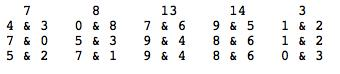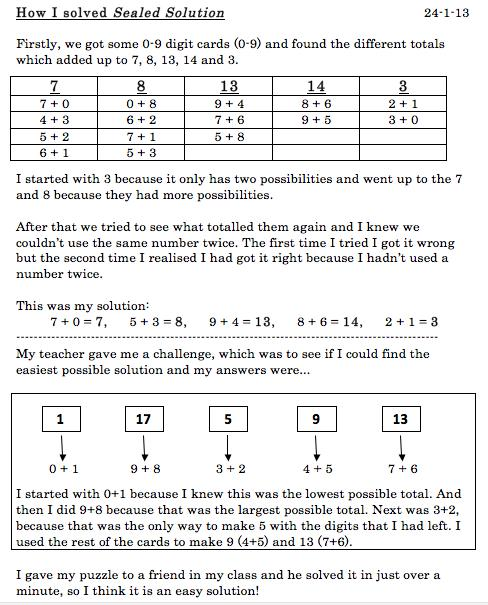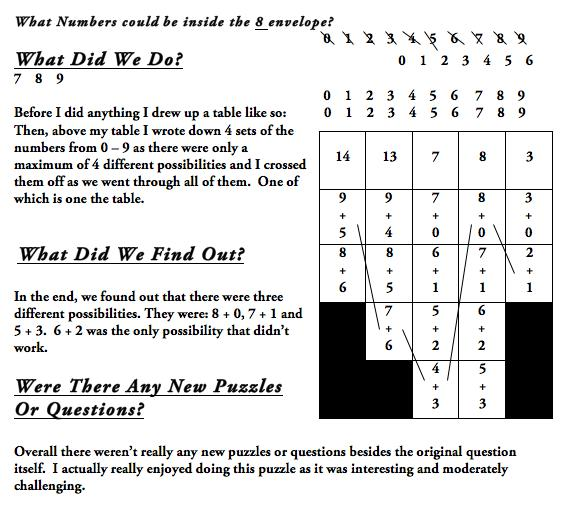#### You may also like### Consecutive Numbers

An investigation involving adding and subtracting sets of consecutive numbers. Lots to find out, lots to explore.### Roll These Dice

Roll two red dice and a green dice. Add the two numbers on the red dice and take away the number on the green. What are all the different possible answers?### Domino Square

Use the 'double-3 down' dominoes to make a square so that each side has eight dots.

# Sealed Solution

##### Age 7 to 11 Challenge Level:

We had well over 200 solutions sent in to this activity, the most we've ever had to read! Thank you all, there were lots that made interesting reading, particularly from those who described how they went about working on the challenge. The solutions also came from many parts of the world: the Czech Republic, United Arab Emirites, the United Kingdom, the United States of America and Australia. We are sorry we cannot include all the correct solutions but here are a few that give you a taste of how it was approached.

Year 6 pupils from St John Fisher Harrogate Magic Maths Club:

We started off by thinking of all the possible ways of making the totals. This took a long time.
We thought that it would be best to make the biggest totals first, using the bigger numbers to make them:
14 = 9 + 5, 13 = 6 + 7, 1 + 2 = 3, 4 + 3 = 7 and 8 + 0 = 8.
Some of us did it the other way round, making the smallest totals first, with the smallest numbers:
1 + 2 = 3, 4 + 3 = 7, 8 + 0 = 8, 7 + 6 = 13 and 9 + 5 = 14.
We could also come up with pairs randomly but it's quicker to use a strategy.
7 + 0 = 7, 5 + 3 = 8, 9 + 4 = 13, 6 + 8 = 14 and 1 + 2 = 3.

Ieuan from Riverside School, Prague in the Czech Republic wrote:

At first I was randomly picking numbers, and on my first attempt doing it I found a solution:
0 + 7=7 5 + 3=8 9 + 4 =13 6 + 8=14 2 + 1=3.
And then I tried using a system from then on, of adding a number to the smaller number then subtracting one from the smaller number, but it did not go very well because when I converted 5 + 3 to 4 + 4 I realised that you cannot do that.
Then I found out something quite clever that from one onward: each 2 numbers have the same amount of possibilities (I think by this Ieuan means that pairs of consecutive numbers have the same number of possibilities).  For example 2 and 3 have two possibilities, 4 and 5 have have three, 6 and 7 have four, 8 and 9 have five and it goes on forever! So I wrote down all the possibilities for 7, 8, 13, 14, 3.
Then I shortened it, so if I use 13 as an example: 13+0, 12+1, 10+3, 9+4, 8+5, 7+6. Then I would take off 13+0, 12+1 and 10+3 and do that for all the rest! So when I had all the possibilities I did two attempts without succeding then I got one and I started explaining it on here, but I realised I had found the same as my first attempt.
Then I did one attempt and I found another:   3+0, 8+6, 9+4, 7+1 and 2+5.

Ellie in Year 5 at St. Nicolas CE Junior School, Newbury.

I found three solutions:For my second solution, I tried a different solution for 14 which was 8 and 6. Then for 13 I had to use 9 and 4. There were two solutions to make 8 and this time I chose 5 and 3. Then 7 was made from 0 and 7 and 3 from 1 and 2 which all worked.
For my last solution, I used 8 and 6 for 14 again and 9 and 4 for 13. For 8, I used 7 and 1 which was different. To make 7, I used 5 and 2 (also different) and for 3, I used 0 and 3.
There were no other ways of making 14 so I knew I had found all the solutions.

So the numbers which could be inside the 8 envelope were 0 & 8, 5 & 3 or 7 & 1.

Rebekah from Thameside Primary School sent in a Word Document as follows:From Angus  of Canberra Grammar School Australia we had another interesting way of approaching this kind of problemThank you again for sharing all your solutions.